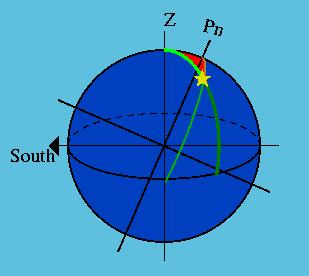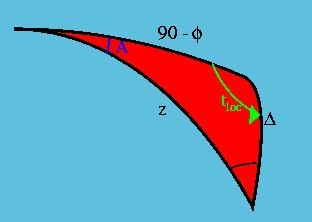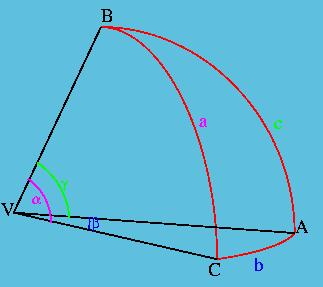Astronomical Triangle

# Astronomical Triangle

1. The Celestial Sphere
2. The Astronomical Triangle
3. Lines of Position
4. References and Postscript CodeFigure 5a

When drawn on the same sphere the verticle circle of the body and the celestial meridian of the body form a spherical triangle (shown in red in Figure 5a above). The three corners are the zenith (Z), the elevated pole, and the location of the body on the celestial sphere. Figure 5b below is an enlarged version of this triangle commonly called the Astronmical Triangle.Figure 5b

In the above Figure, the angle A is the azimuth of the body. Remember that the azimuth is either the arc or the spherical angle between the observer's meridian and the vertical circle of the body. In this case it is represented as the spherical angle of the arc, drawn at the observer's zenith. The other angle that we will use is the tloc, the local hour angle of the body. The local hour angle is the spherical angle between the observer's meridian and the body's meridian, drawn here at the elevated pole.

The 3 sides of the triangle are z the zenith distance, D the polar distance, and 90-f, which is often called the colatitude, and will be denoted col from here on.

col = 90 - f

So now the question is..."How can we solve the astronomical Triangle?"Figure 6

Figure 6 shows a spherical triangle drawn on the surface of a sphere of arbitray radius R:

VC = VB = VA = R

The arclength of each side is a,b and c, with angles respectively of a, b and g. An angle between two planes is known as a dihedral angle. A dihedral angle is calculated by taking the dot product of the normal of each plane. The normal of a plane is given by the cross product of two vectors in the plane. For example the normals of planes BVA and CVA are:
BVAn = VAo x VBo
CVAn = VAo x VCo
where the o subscript means that these vectors have been normalized. From this we can find the dihedral angle of planes BVA and CVA as:
(VAo x VBo).(VAo x VCo) = (|VAo||VBo|sinc)(|VAo||VCo|sinb)cosA
however the lengths of normalized vectors are always unity, hence:
(VAo x VBo).(VAo x VCo) = sincsinbcosA   *
Now using the cross product identity, and allowing A B and C to be the angles at the vertices inside the triangle as well as the vertices we find that:
(VAo x VBo).(VAo x VCo) = VAo.[VBo x (VAo x VCo)]
= VAo.[VAo(VBo.VCo) - VCo(VAo.VBo)]
= (VBo.VCo) - (VAo.VCo)(VAo.VBo)
= cosa - cosbcosc   **
equations * and ** are both equal to the same dihedral angle, and so setting them equal to each other gives:

cosa = cosbcosc + sinbsinccosA

This is the "Law of Cosines" for a spherical triangle.

Using the astronmical triangle already established and the law of cosines, we have two equations:

cosz = cos(col)cosD + sin(col)sinDcos(tloc)
cosD = coszcos(col) + sinzsin(col)cosZ

With the aid of the trig identity cos(90-x) = sinx and the definitions of col, tloc, z, and D these become:

sinh = sin(f)sind + cos(f)cosdcos(l - GHA )           (1)

sind = sin(h)sin(f) + cos(h)cos(f)cosA       (2)

These equations are known as the "Sight Reduction Formulas". If A, d, GHA, and h are known, then these two equations can be solved for f and l. However the process is elaborate requiring many iterations to reach a good solution. With a computer it is fairly easy and quick and is often used on well equipped ships to navigate. However for those of us without a computer resource there is another method to determine our location that doesn't involve solving the astronomical triangle.

Top of page
The Celestial Sphere
Lines of Position
References and Postscript Code